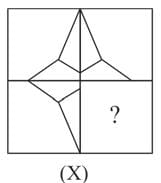Olympiad Test: Completion Of Incomplete Pattern - 1

# Olympiad Test: Completion Of Incomplete Pattern - 1

Test Description

## 10 Questions MCQ Test Mathematical Olympiad Class 8 | Olympiad Test: Completion Of Incomplete Pattern - 1

Olympiad Test: Completion Of Incomplete Pattern - 1 for Class 8 2022 is part of Mathematical Olympiad Class 8 preparation. The Olympiad Test: Completion Of Incomplete Pattern - 1 questions and answers have been prepared according to the Class 8 exam syllabus.The Olympiad Test: Completion Of Incomplete Pattern - 1 MCQs are made for Class 8 2022 Exam. Find important definitions, questions, notes, meanings, examples, exercises, MCQs and online tests for Olympiad Test: Completion Of Incomplete Pattern - 1 below.
 1 Crore+ students have signed up on EduRev. Have you?
Olympiad Test: Completion Of Incomplete Pattern - 1 - Question 1

### Direction: In the following problems, select a figure from amongst the four options which when placed in the blank space of figure (X) would complete the pattern.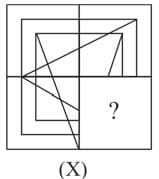Olympiad Test: Completion Of Incomplete Pattern - 1 - Question 2

### Direction: In the following problems, select a figure from amongst the four options which when placed in the blank space of figure (X) would complete the pattern.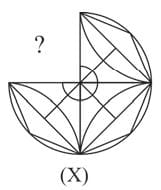Olympiad Test: Completion Of Incomplete Pattern - 1 - Question 3

### Direction: In the following problems, select a figure from amongst the four options which when placed in the blank space of figure (X) would complete the pattern.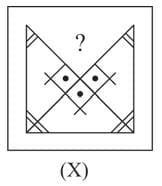Olympiad Test: Completion Of Incomplete Pattern - 1 - Question 4

Direction: In the following problems, select a figure from amongst the four options which when placed in the blank space of figure (X) would complete the pattern.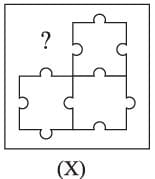Olympiad Test: Completion Of Incomplete Pattern - 1 - Question 5

Direction: In the following problems, select a figure from amongst the four options which when placed in the blank space of figure (X) would complete the pattern.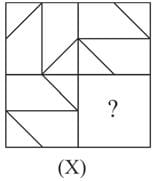Olympiad Test: Completion Of Incomplete Pattern - 1 - Question 6

Direction: In the following problems, select a figure from amongst the four options which when placed in the blank space of figure (X) would complete the pattern.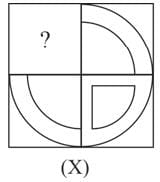Olympiad Test: Completion Of Incomplete Pattern - 1 - Question 7

Direction: In the following problems, select a figure from amongst the four options which when placed in the blank space of figure (X) would complete the pattern.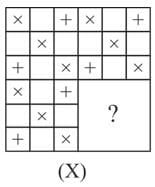Olympiad Test: Completion Of Incomplete Pattern - 1 - Question 8

Direction: In the following problems, select a figure from amongst the four options which when placed in the blank space of figure (X) would complete the pattern.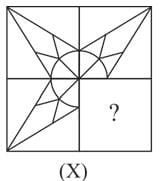Olympiad Test: Completion Of Incomplete Pattern - 1 - Question 9

Direction: In the following problems, select a figure from amongst the four options which when placed in the blank space of figure (X) would complete the pattern.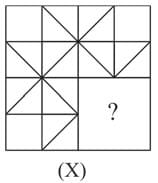Olympiad Test: Completion Of Incomplete Pattern - 1 - Question 10

Direction: In the following problems, select a figure from amongst the four options which when placed in the blank space of figure (X) would complete the pattern.